Marin Mersenne was a French mathematician and music theorist and through his 1637 work Traité del l'harmonie we can understand the relationship between frequency of a string and its length, weight, and tension. Mersenne established the following three relationships:

• The frequency of a string is inversely proportional to twice its length. Hence, the shorter the string, the higher the frequency. So if we double the string length, L, then we get half the frequency, f.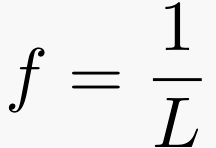• The frequency of a string is directly proportional to the square root of its tension, F. Thus, the tension is equal to the frequency squared. This means that with a higher tension, the frequency will also be higher, so if we double the tension, then the frequency is quadrupled.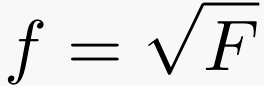• The frequency is inversely proportional to the square root of the mass-per-unit-length, W. Thus, the smaller the mass of the string, the higher the frequency. A lighter string with the same tension will have a higher pitch than a heavier string. If we quadruple the mass of the string, it will cut the frequency in half.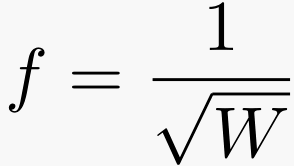• These three relationships can be summed into one equation, which is known as Mersenne's Law: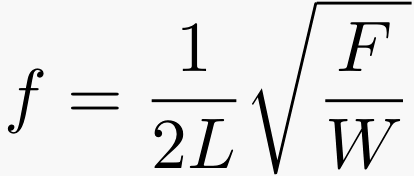• Knowing these relationships and formulas, we can calculate mass-per-unit-length, tension, and diameter by the following three equations: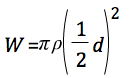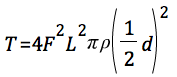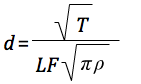Now that we know these relationships, how does it relate to the double bass? Mersenne's Law shows that if you want a lower pitch, you want a thicker, heavier, low tension string. On the other hand, if you want a high pitch, you want a thin, light, high tension string. This proves true on the bass; the E string, the string with the lowest pitch, is also the heaviest, thickest, and has the least amount of tension, while the opposite is true of the G string which has the highest pitch. A double bass is tuned by twisting the metal tuners within the pegbox. What this is really doing from the physics standpoint is increasing or decreasing the tension in the string depending on which way you turn the tuners, which then plays a higher pitch if the tension is increased and a lower pitch when tension is decreased.

Also, the note that is played is determined by pressing down on the string at a certain place on the fingerboard. When doing this, the player is actually decreasing the length of the string that is free to vibrate. Thus, a note played near the pegbox will have a much lower pitch than one played close to the bridge. This is the reason why notes get higher as you move away from the pegbox and towards the bridge.

Photos from: Essentials of Physics